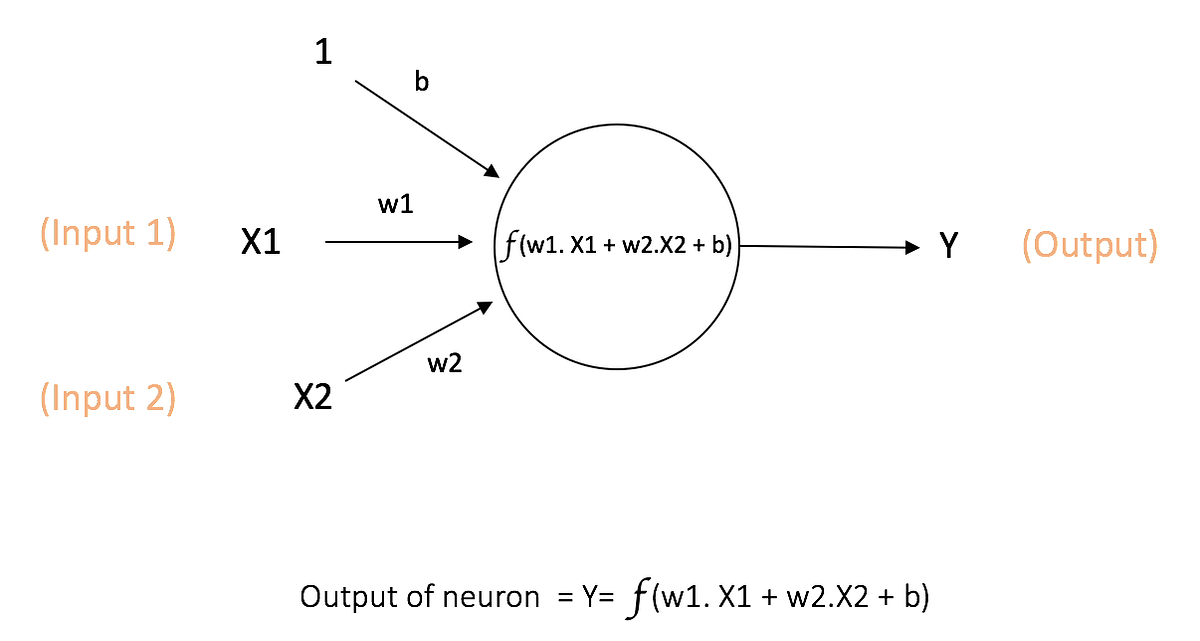# Introduction to Neural NetworksThere has been hype about artificial intelligence, machine learning, and neural networks for quite a while now. This will not be a math-heavy introduction because I just want to build the idea here.

There has been hype about artificial intelligence, machine learning, and neural networks for quite a while now. I have been working on these things for over a year now so I would like to share some of my knowledge and give my point of view on Neural networks. This will not be a math-heavy introduction because I just want to build the idea here.

I will start from the neural network and then I will explain every component of a neural network. If you feel like something is not right or need any help with any of this, Feel free to contact me, I will be happy to help.

## When to use the Neural Network?

Let’s assume we want to solve a problem where you are given some set of images and you have to build an automated system that can categories each of those images to its correct label.

The problem looks simple but how do we come with some logic using raw pixel values and target labels. We can try comparing pixels and edges but we won’t be able to come with some idea which can do this task effectively or say the accuracy of 90% or more.

When we have this kind of problem where we have high dimensional data like Images and we don’t know the relationship between Input(Images) and the Output(Labels), In this kind of scenario we should use Neural Networks.ư

## What is the Neural network?

Artificial neural networks, usually simply called neural networks, are computing systems vaguely inspired by the biological neural networks that constitute animal brains. An ANN is based on a collection of connected units or nodes called artificial neurons, which loosely model the neurons in a biological brain

## ML Optimization pt.1 - Gradient Descent with Python

In this article, we explore gradient descent - the grandfather of all optimization techniques and it’s variations. We implement them from scratch with Python.

## Artificial Neural Network | Deep Learning with TensorFlow and Artificial Intelligence

Artificial Neural Network | Deep Learning with Tensorflow and Artificial Intelligence | I have talked about Artificial neural networks and its implementation in TensorFlow using google colab. You will learn: What is an Artificial Neural Network? Building your neural network using Tensorflow.

## Deep Learning 101 —  Neural Networks Explained

The past few decades have witnessed a massive boom in the penetration as well as the power of computation, and amidst this information.

## Deep Learning Explained in Layman's Terms

Deep Learning Explained in Layman's Terms. In this post, you will get to learn deep learning through a simple explanation (layman terms) and examples.

## Artificial Intelligence, Machine Learning, Deep Learning

Artificial Intelligence (AI) will and is currently taking over an important role in our lives — not necessarily through intelligent robots.